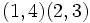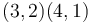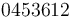# Foata transformation

## Definition

Suppose$X$ is a totally ordered finite set of size$n$. We denote by$\operatorname{Sym}(X)$ the symmetric group on$X$. The typical case is that$X = \{ 1, 2, \dots, n\}$, but we sometimes want to use other labels, specifically other contiguous blocks of integers. In the case that$X = \{ 1, 2, \dots, n\}$, we call the group$S_n$.

The Foata transformation, also called the Foata correspondence or Foata fundamental transformation, is a bijection from the symmetric group$\operatorname{Sym}(X)$ to itself described below. The transformation essentially relies on "punning" between the canonical notation for the cycle decomposition of a permutation and the one-line notation of the permutation being mapped to.

The correspondence hinges on the choice of total ordering on the set and is not invariant under group conjugations, nor is it a group homomorphism. Its significance is more combinatorial than algebraic.

### Forward direction

Start with a permutation$\sigma \in \operatorname{Sym}(X)$.

1. Write the permutation in canonical cycle decomposition notation: By the cycle decomposition theorem, the permutation has a cycle decomposition. Write the cycle decomposition in canonical notation, including fixed points. Make sure to include fixed points as cycles of size one. Explicitly, this means:
• Cyclically rearrange each cycle so that it begins with its largest element.
• Arrange the cycles in increasing order of their largest elements.
2. Reinterpret in one-line notation: Now, drop the commas and parentheses and you get a string of length$n$ that is the one-line notation for a permutation of$X$ (more explicitly, it is the second line of the two-line notation of the permutation of$X$, where the first line is the elements of$X$ in ascending order with respect to their total order). This is the new permutation that is the image of the Foata transformation.

### Reverse direction

The above two steps describe how to start with a permutation and apply the Foata transformation to it to obtain a new permutation. Here, we describe how to reverse the process, i.e., how to go from the image of a permutation under the Foata transformation back to the original permutation. The steps are:

1. Write the permutation in one-line notation (i.e., write it as a string whose$i^{th}$ element is the image of the$i^{th}$ element of$X$ under the permutation).
2. Traverse through the string from left to right, and identify all the left-to-right maxima, i.e., all the elements that are larger than the elements before them. The strings starting with a given left-to-right maxima and ending just before the next form the strings for the cycles in the canonical notation for the cycle decomposition of the original permutation.

#### Replacing largest with smallest

There are conflicting definitions of the canonical notation. Some definitions require that we use the smallest element, and decreasing order. Explicitly, they suggest that:

• We cyclically rearrange each cycle so that it begins with its smallest element.
• We order the cycles in decreasing order of their smallest elements.

The definitions are equivalent up to some other transformations, and are not conceptually too far apart, though there are cases where one definition is a little more useful than the other. We stick with the definition using largest elements, because then the Foata transformation fixes the identity element of the symmetric group.

## Examples

### Forward direction

#### Example 1

Consider the permutation of the set$\{1,2,3,4,5 \}$ with one-line notation:$3 1 2 5 4$

We first write this in cycle decomposition:$(1,3,2)(4,5)$

We now rewrite in canonical notation:$(3,2,1)(5,4)$

We now drop the parentheses and obtain the one-line notation for the transformed permutation:$3 2 1 5 4$

#### Example 2

Consider the permutation of the set$\{ 0,1,2,3,4,5,6 \}$ that sends every element to its fifth power modulo 7. The one-line notation for this permutation is:$0 1 4 5 2 3 6$

The cycle decomposition of this permutation is:$(0)(1)(2,4)(3,5)(6)$

Rearranging each cycle to begin with its largest element and arranging the cycles in increasing order of their largest element, we obtain:$(0)(1)(4,2)(5,3)(6)$

Dropping parentheses, we get:$0 1 4 2 5 3 6$

#### Example 3

Consider the permutation on$\{ 1,2,3,4\}$ given as$x \mapsto 5 - x$. In one-line notation, the permutation is:$4 3 2 1$

The permutation has the cycle decomposition:$(1,4)(2,3)$

In canonical notation, this becomes:$(3,2)(4,1)$

Dropping the parentheses, we get:$3 2 4 1$

### Reverse direction

#### Example 1

Consider a permutation of the set$\{ 0,1,2,3,4,5,6 \}$ that multiplies everything by 3 mod 7. First, we write this in one-line notation:$0 3 6 2 5 1 4$

Now, we identify the left-to-right maxima. They occur at 0, 3, and 6. The cycles of the new permutation are therefore (0), (3), and (6, 2, 5, 1, 4). The new permutation is therefore the following in cycle decomposition notation:$(0)(3)(6, 2, 5, 1, 4)$

Dropping fixed points, we get a single 5-cycle:$(6,2,5,1,4)$

In one-line notation, the new permutation is:$0 4 5 3 6 1 2$

#### Example 2

Consider the permutation of the set$\{ 1,2,3,4,5,6,7,8 \}$ given in one-line notation as follows:$3 1 5 2 4 7 6 8$

The left-to-right maxima are 3, 5, 7, and 8, and the cycle decomposition is therefore:$(3,1)(5,2,4)(7,6)(8)$

In one-line notation, this becomes:$3 4 1 5 2 7 6 8$

## Behavior

### Compatibility with maps of symmetric groups

The symmetric groups form an IAPS of groups, and explicitly, there are maps:$S_m \times S_n \to S_{m + n}$

where the image of a pair of permutations is obtained by multiplying the first permutation on$\{ 1,2,\dots, m \}$ with the second permutation applied to the set$\{ m+1,\dots, m+n \}$ (i.e., changing label$i$ to label$m + i$.

The Foata transformation behaves nicely with respect to this homomorphism. Explicitly, if we denote the map above as$\varphi_{m,n}$, and the Foata transformations as$\tau_m, \tau_n, \tau_{m+n}$ respectively, then:$\varphi_{m,n}(\tau_m(x), \tau_n(y)) = \tau_{m+n}(\varphi_{m,n}(x,y))$

### Fixed points

Based on the above compatibility observation, and the fact that the Foata transformation is the identity map on the symmetric groups of size one and two, we obtain that the Foata transformation's fixed points include all permutations whose cycle decomposition comprises fixed points and transpositions between adjacent elements (in terms of the description of the symmetric group as a Coxeter group, these transpositions are the generators, so we're really talking here about elements that are products of pairwise commuting elements of the Coxeter generating set). In fact, it can be proved that these are the only fixed points.

The number of such fixed points in$\operatorname{Sym}(X)$, for$X$ of size$n$, is:$\sum_{k=0}^{\lfloor n/2 \rfloor} \binom{n - k}{k}$

where the value for a given$k$ is the number of fixed points that are products of$k$ pairwise disjoint transformations.

It can also be proved that this sum equals the Fibonacci number$F_{n+1}$, and in particular, this means that the value for a given$n$ equals the sum of the values for the two preceding values of$n$. There are two ways of proving it:

• Proving it from the product of transpositions description: The inductive step (showing that the value at$n$ is the sum of the values at$n - 1$ and$n - 2$) follows by making cases on whether the largest element is fixed. The base case involves a simple examination of the cases$n = 1, n = 2$.
• Direct algebraic manipulation of the closed form expression above, using combinatorial identities satisfied by the binomial coefficients.
Value of$n$ Number of fixed points List of fixed points in one-line notation
1 1 1
2 2 12, 21
3 3 123, 213, 132
4 5 1234, 2134, 1324, 1243, 2143
5 8 12345, 21345, 13245, 12435, 12354, 21435, 21354, 13254